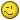# EXCEL.EXE process does not end after adding an excel chart

M

#### marcnikko

Hi,

I have VB .NET function below that creates an excel document. It
works fine and the Excel.exe process ends except when I try to add a
chart. When I include the code here I cannot cleanly end the Excel.exe
process:

xlChart.ChartType = Excel.XlChartType.xlXYScatterLines

Once I include that code the EXCEL.EXE process is still in the task
manager.

What can I do to close the EXCEL.EXE process?

Thanks,

Marc

Code:

Imports Excel = Microsoft.Office.Interop.Excel

Function CreateExcelPlot() As String
Dim plotFilePath As String = Nothing
Dim xlApp As Excel.Application
Dim xlBook As Excel.Workbook
Dim xlDataSheet As Excel.Worksheet
Dim xlChartSheet As Excel.Worksheet
Dim xlChart As Excel.Chart

'Initiate Excel, hide the application, and add a workbook. In
case you were wondering, by referring to the Excel
'application, the program is initiated
xlApp = New Excel.Application
xlApp.Visible = False
xlChart.ChartType = Excel.XlChartType.xlXYScatterLines 'Once
I include this, I can't cleanly close EXCEL.EXE

xlDataSheet.Cells(1, 1) = DateValue("January 1, 2003")
xlDataSheet.Cells(1, 2) = 30
xlDataSheet.Cells(2, 1) = DateValue("February 1, 2003")
xlDataSheet.Cells(2, 2) = 35
xlDataSheet.Cells(3, 1) = DateValue("March 1, 2003")
xlDataSheet.Cells(3, 2) = 31
xlDataSheet.Cells(4, 1) = DateValue("April 1, 2003")
xlDataSheet.Cells(4, 2) = 36
'xlChart.SetSourceData(xlDataSheet.Range("A1:B4")) Ignore for
now

plotFilePath = "C:\Test13.xls"

Try
My.Computer.FileSystem.DeleteFile(plotFilePath)
Catch
End Try

xlBook.SaveAs(plotFilePath)

xlBook.Close()
xlApp.Workbooks.Close()
xlApp.Quit()
System.Runtime.InteropServices.Marshal.ReleaseComObject(xlApp)
System.Runtime.InteropServices.Marshal.ReleaseComObject
(xlDataSheet)
System.Runtime.InteropServices.Marshal.ReleaseComObject
(xlChartSheet)
System.Runtime.InteropServices.Marshal.ReleaseComObject
(xlChart)
System.Runtime.InteropServices.Marshal.ReleaseComObject
(xlBook)
xlApp = Nothing
xlBook = Nothing
xlDataSheet = Nothing
xlChartSheet = Nothing
xlChart = Nothing
GC.Collect()
return plotFilePath
End Function

J

#### Jim Cone

Marc,
I don't speak VB.NET but I have some comments/suggestions.
(you get what you pay for) <g>
Your complaint is most ofter heard (speaking VBA) when orphan references
are created and they are not Set to nothing before exiting....

1. Use xlApp instead of Excel...
xlChart.ChartType = xlApp.XlChartType.xlXYScatterLines
2. Clean up your child objects first and proceed up the line...
xlChart = Nothing
xlChartSheet = Nothing
xlDataSheet = Nothing
xlBook.Close()
xlBook = Nothing
xlApp.Workbooks.Close()
xlApp.Quit()
xlApp = Nothing
3. Don't quit the app until you have cleaned things up
--
Jim Cone
Portland, Oregon USA

"marcnikko" <[email protected]>
wrote in message
Hi,
I have VB .NET function below that creates an excel document. It
works fine and the Excel.exe process ends except when I try to add a
chart. When I include the code here I cannot cleanly end the Excel.exe
process:

xlChart.ChartType = Excel.XlChartType.xlXYScatterLines

Once I include that code the EXCEL.EXE process is still in the task
manager.
What can I do to close the EXCEL.EXE process?
Thanks,
Marc

Code:
Imports Excel = Microsoft.Office.Interop.Excel

Function CreateExcelPlot() As String
Dim plotFilePath As String = Nothing
Dim xlApp As Excel.Application
Dim xlBook As Excel.Workbook
Dim xlDataSheet As Excel.Worksheet
Dim xlChartSheet As Excel.Worksheet
Dim xlChart As Excel.Chart

'Initiate Excel, hide the application, and add a workbook. In
case you were wondering, by referring to the Excel
'application, the program is initiated
xlApp = New Excel.Application
xlApp.Visible = False
'Once I include this, I can't cleanly close EXCEL.EXE
xlChart.ChartType = Excel.XlChartType.xlXYScatterLines

xlDataSheet.Cells(1, 1) = DateValue("January 1, 2003")
xlDataSheet.Cells(1, 2) = 30
xlDataSheet.Cells(2, 1) = DateValue("February 1, 2003")
xlDataSheet.Cells(2, 2) = 35
xlDataSheet.Cells(3, 1) = DateValue("March 1, 2003")
xlDataSheet.Cells(3, 2) = 31
xlDataSheet.Cells(4, 1) = DateValue("April 1, 2003")
xlDataSheet.Cells(4, 2) = 36
'xlChart.SetSourceData(xlDataSheet.Range("A1:B4")) Ignore for now
plotFilePath = "C:\Test13.xls"
Try
My.Computer.FileSystem.DeleteFile(plotFilePath)
Catch
End Try
xlBook.SaveAs(plotFilePath)

xlBook.Close()
xlApp.Workbooks.Close()
xlApp.Quit()
System.Runtime.InteropServices.Marshal.ReleaseComObject(xlApp)
System.Runtime.InteropServices.Marshal.ReleaseComObject
(xlDataSheet)
System.Runtime.InteropServices.Marshal.ReleaseComObject
(xlChartSheet)
System.Runtime.InteropServices.Marshal.ReleaseComObject
(xlChart)
System.Runtime.InteropServices.Marshal.ReleaseComObject
(xlBook)
xlApp = Nothing
xlBook = Nothing
xlDataSheet = Nothing
xlChartSheet = Nothing
xlChart = Nothing
GC.Collect()
return plotFilePath
End Function

B

#### Barb Reinhardt

You forgot to include "Set" to set your objects to Nothing.C

#### Chip Pearson

You forgot to include "Set" to set your objects to Nothing.In VB.NET, there is no "Set" keyword since everything is basically an
object already. VS2005 and earlier would automatically remove the Set
keyword if you used it in an assignment, but VS2008+ doesn't do this.

Cordially,
Chip Pearson
Microsoft Most Valuable Professional
Excel Product Group, 1998 - 2009
Pearson Software Consulting, LLC
www.cpearson.com
(email on web site)

M

#### marcnikko

I solved this issue using your guys suggestions. For others
encountering similar issues here are some guidelines I learned the
hard way.

1) Declare every object
2) Cleanup your objects in the correct order

Here's the code that works:

Function CreateExcelPlot() As String
Dim plotFilePath As String = Nothing
Dim xlApp As Excel.Application
Dim xlWorkBooks As Excel.Workbooks
Dim xlBook As Excel.Workbook
Dim xlDataSheet As Excel.Worksheet
Dim xlChart As Excel.Chart
Dim xlChartRange As Excel.Range

'Initiate Excel, hide the application, and add a workbook. In
case you were wondering, by referring to the Excel
'application, the program is initiated
xlApp = New Excel.Application
xlApp.Visible = False
xlWorkBooks = xlApp.Workbooks
xlChart.ChartType = Excel.XlChartType.xlXYScatterLines

xlDataSheet.Cells(1, 1) = DateValue("January 1, 2003")
xlDataSheet.Cells(1, 2) = 30
xlDataSheet.Cells(2, 1) = DateValue("February 1, 2003")
xlDataSheet.Cells(2, 2) = 35
xlDataSheet.Cells(3, 1) = DateValue("March 1, 2003")
xlDataSheet.Cells(3, 2) = 31
xlDataSheet.Cells(4, 1) = DateValue("April 1, 2003")
xlDataSheet.Cells(4, 2) = 36

xlChartRange = xlDataSheet.Range("A1:B4")
xlChart.SetSourceData(xlChartRange)

plotFilePath = "C:\Test13.xls"

Try
My.Computer.FileSystem.DeleteFile(plotFilePath)
Catch
End Try

xlBook.SaveAs(plotFilePath)

'Cleanup so Excel closes
System.Runtime.InteropServices.Marshal.ReleaseComObject
(xlChartRange)
xlChartRange = Nothing
System.Runtime.InteropServices.Marshal.ReleaseComObject
(xlChart)
xlChart = Nothing
System.Runtime.InteropServices.Marshal.ReleaseComObject
(xlDataSheet)
xlDataSheet = Nothing
xlBook.Close()
System.Runtime.InteropServices.Marshal.ReleaseComObject
(xlBook)
xlBook = Nothing
xlWorkBooks.Close()
System.Runtime.InteropServices.Marshal.ReleaseComObject
(xlWorkBooks)
xlWorkBooks = Nothing
xlApp.Quit()
System.Runtime.InteropServices.Marshal.ReleaseComObject(xlApp)
xlApp = Nothing
GC.Collect()
GC.WaitForPendingFinalizers()
Return plotFilePath
End Function